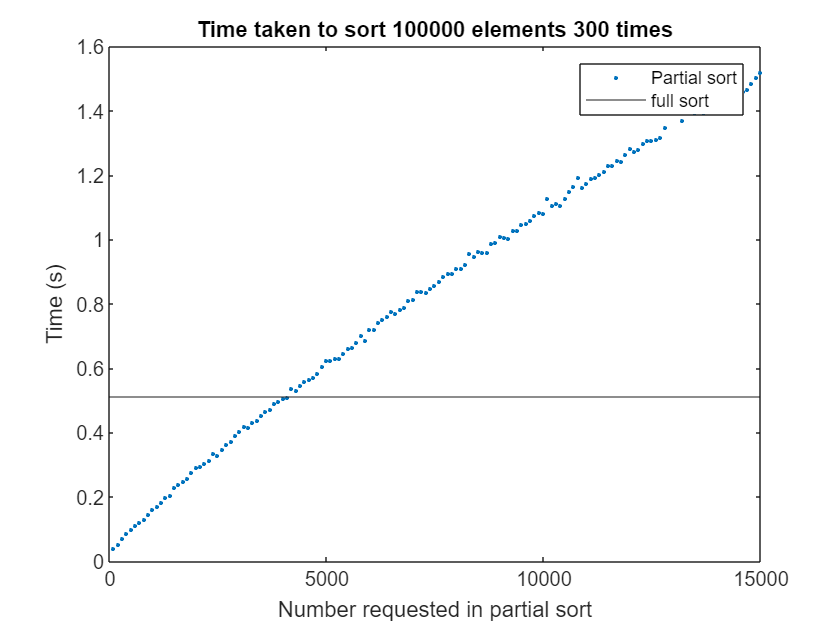# Partial sorting in MATLAB

I was recently working with a researcher who had to sort arrays of double precision numbers thousands of times. In his application, however, he didn't need the full sorted list. He only wanted the lowest N/2 elements of the array sorted. As such, he had two questions.
• Does MATLAB have partial sort functions?
• Will they be faster for his application?
The answer to the first question is easy. MATLAB has mink(x,k) and maxk(x,k) which find the lowest or highest sorted k elements from the array x respectively.
The answer to the second question "Will partial sorting be faster?" depends on k as you might expect. Let's investigate how

## How long does a full sort take compared to a partial sort requesting the smallest 10 entries?

We create a 100,000 element array of doubles
x = rand(100000,1);
I'm going to sort this array 300 times to match how it's used in the user's application. Of course, in the real application, x varies every iteration but I want to isolate just the sort
tic
for i=1:300
y = sort(x);
res = y(1:10); % We only want the lowest 10
end
sortTime=toc
sortTime = 0.5181
If all we want is the 10 smallest numbers in x sorted, we can alternatively use mink
tic
for i=1:300
res = mink(x,10);
end
partialsortTime=toc
partialsortTime = 0.0361
fprintf("Partial sort is %fx faster than full sort for k=10",sortTime/partialsortTime)
Partial sort is 14.354572x faster than full sort for k=10
So, as you might expect, partial sorting is very useful when you only want a small number of sorted elements from a large array

## Is partial sorting always faster than full sorting?

Next, I use the mink function to request the lowest k numbers where k varies from 100 to 15000, As before, I repeat each sort operation 300 times.
minkResults = zeros(150,1);
minkSize = zeros(150,1);
for j=1:150
tic
for i=1:300
y=mink(x,j*100);
end
minkResults(j)=toc;
minkSize(j)=j*100;
end
Plotting the results, we see that a partial sort is only faster than a full sort if we are requesting fewer than 4200 elements -- that's less than 5% of the full vector.
plot(minkSize,minkResults,'.')
yline(sortTime)
legend("Partial sort", "full sort")
xlabel("Number requested in partial sort")
ylabel("Time (s)")
title("Time taken to sort " + numel(x) + " elements 300 times")crossing = minkSize(find(minkResults>sortTime,1,"first"));
fprintf("For x of size %d, mink(x,k) is faster than sort(x) only if k < %d}",numel(x),crossing)
For x of size 100000, mink(x,k) is faster than sort(x) only if k < 4200}

## Why isn't partial sorting better?

You may be disappointed with this result because, for this example at least, partial sorting is only the better option if you want fewer than 5% of the total number of elements sorted. The primary reason for this is that MATLAB's sort function is both fast and multithreaded. It's pretty hard to beat!
Let's take a look at how sort scales with 100,000 elements. I'll repeat the computation 300 times to get a stable average.
% Scaling of sort with respect to threads
arraySize = 100000;
repeats = 300;
sortSpeedTest(arraySize,repeats)
ans = 4×3 table
110.00521
220.00281.8151
340.00182.8250
480.00153.5265
The algorithm used in the mink function, on the other hand, does not lend itself to multithreading. As such, it is completely single threaded. Let's see this explicity using the same sized input matrix, requesting the lowest 10,000 numbers be sorted.
%Scaling of mink with respect to threads
k=10000;
minkSpeedTest(arraySize,repeats,k)
ans = 4×3 table
110.00371
220.00370.9967
340.00371.0018
480.00361.0197
It's pretty clear that mink doesn't benefit from differing numbers of threads. Any differences we see are just measurement noise. If sort(x) were not mulithreaded, mink(x,k) would be faster here but multithreading helps a lot.
As we increase k, there will eventually come a point where even comparing single threaded sort with mink results in mink losing. On my machine, k=16000 does it
%Scaling of mink with respect to threads
k=16000;
minkSpeedTest(arraySize,repeats,k)
ans = 4×3 table
110.00531
220.00531.0141
340.00540.9917
480.00531.0129
Slightly slower than a single threaded full sort! A possible future enhacement to MATLAB's mink function would be to switch to using sort internally for large k since, sooner or later, mink's algorithm just isn't the right choice.

You will probably have different results from me if you run these tests on your machine. As such, some information about my machine may be useful. Here, I use the excellent cpuinfo function by Ben Tordoff, available on the MATLAB File Exchange.
cpuinfo
ans = struct with fields:
CPUName: '11th Gen Intel(R) Core(TM) i7-11700 @ 2.50GHz' Clock: '2501 MHz' Cache: 4194304 TotalMemory: 3.4010e+10 NumCPUs: 1 TotalCores: 8 OSType: 'Windows' OSVersion: 'Microsoft Windows 11 Pro' Hostname: 'DESKTOP-N3C7C6Q'

## Over to you

In summary, partial sorting in MATLAB can be useful but only when used appropriately. Have you used mink or maxk in your work...or have you just discovered them now? What additional sorting functionality do you think MATLAB should have?

### Helper functions

function result = sortSpeedTest(arraySize,repeats)
% Runs sort on an array of size arraySize using differing numbers of threads
format short
averageTime = zeros(4,1);
SpeedUp = zeros(4,1);
x = rand(1,arraySize);
for testNum = 1:4
tic
for i=1:repeats
y = sort(x);
end
averageTime(testNum) = toc/repeats;
SpeedUp(testNum) = averageTime(1)/averageTime(testNum);
end
end

function result = minkSpeedTest(arraySize,repeats,k)
% Runs mink on an array of size arraySize using differing numbers of threads
format short
averageTime = zeros(4,1);
SpeedUp = zeros(4,1);
x = rand(1,arraySize);
for testNum = 1:4
tic
for i=1:repeats
y = mink(x,k);
end
averageTime(testNum) = toc/repeats;
SpeedUp(testNum) = averageTime(1)/averageTime(testNum);
end